Updating search results...

# 11 Results

View
Selected filters:
• Cube
Remix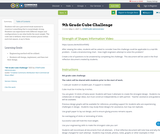Conditional Remix & Share Permitted
CC BY-NC-SA
Rating
0.0 stars

Students will use a perceived weak material to construct something that is surprisingly strong.
Students can experiment with different shapes and configurations to see what holds the most weight.
The cube size is defined, what each student places within each 4x4 square, is up to them.

Subject:
Engineering
Material Type:
Activity/Lab
Lesson
Author:
05/03/2021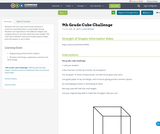Conditional Remix & Share Permitted
CC BY-NC-SA
Rating
0.0 stars

Students will use a perceived weak material to construct something that is surprisingly strong.
Students can experiment with different shapes and configurations to see what holds the most weight.
The cube size is defined, what each student places within each 4x4 square, is up to them.

Subject:
Engineering
Material Type:
Activity/Lab
Lesson
Author:
John Brander
02/28/2019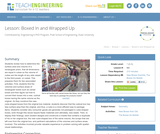Educational Use
Rating
0.0 stars

Students find the volume and surface area of a rectangular box (e.g., a cereal box), and then figure out how to convert that box into a new, cubical box having the same volume as the original. As they construct the new, cube-shaped box from the original box material, students discover that the cubical box has less surface area than the original, and thus, a cube is a more efficient way to package things. Students then consider why consumer goods generally aren't packaged in cube-shaped boxes, even though they would require less material to produce and ultimately, less waste to discard. To display their findings, each student designs and constructs a mobile that contains a duplicate of his or her original box, the new cube-shaped box of the same volume, the scraps that are left over from the original box, and pertinent calculations of the volumes and surface areas involved. The activities involved provide valuable experience in problem solving with spatial-visual relationships.

Subject:
Engineering
Geometry
Material Type:
Activity/Lab
Lesson Plan
Provider:
TeachEngineering
Provider Set:
TeachEngineering
Author:
Mary R. Hebrank
09/18/2014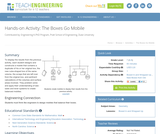Educational Use
Rating
0.0 stars

To display the results from the previous activity, each student designs and constructs a mobile that contains a duplicate of his or her original box, the new cube-shaped box of the same volume, the scraps that are left over from the original box, and pertinent calculations of the volumes and surface areas involved. They problem solve and apply their understanding of see-saws and lever systems to create balanced mobiles.

Subject:
Engineering
Geometry
Material Type:
Activity/Lab
Provider:
TeachEngineering
Provider Set:
TeachEngineering
Author:
Mary R. Hebrank
10/14/2015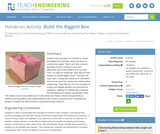Educational Use
Rating
0.0 stars

Student pairs are given 10 minutes to create the biggest box possible using one piece of construction paper. Teams use only scissors and tape to each construct a box and determine how much puffed rice it can hold. Then, to meet the challenge, they improve their designs to create bigger boxes. They plot the class data, comparing measured to calculated volumes for each box, seeing the mathematical relationship. They discuss how the concepts of volume and design iteration are important for engineers. Making 3-D shapes also supports the development of spatial visualization skills. This activity and its associated lesson and activity all employ volume and geometry to cultivate seeing patterns and understanding scale models, practices used in engineering design to analyze the effectiveness of proposed design solutions.

Subject:
Mathematics
Geometry
Measurement and Data
Material Type:
Activity/Lab
Provider:
TeachEngineering
Author:
Aaron Lamplugh
Malinda Zarske
Nathan Coyle
Russell Anderson
Ryan Sullivan
02/07/2017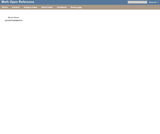Rating
3.0 stars

An interactive applet and associated web page that demonstrate the properties of a cube. A 3-D cube is shown in the applet which can be interactively manipulated using the mouse. Research has shown that some younger students have difficulty visualizing the parts of a 3D object that are hidden. To help with this, the student can rotate the cube in any axis simply by dragging it with the mouse. It can also be 'exploded' - where a slider gradually separates the faces to reveal the ones behind. The cube can also be made translucent so you see through it to the other side. Applet can be enlarged to full screen size for use with a classroom projector, and printed to make handouts. This resource is a component of the Math Open Reference Interactive Geometry textbook project at http://www.mathopenref.com.

Subject:
Geometry
Material Type:
Simulation
Provider:
Math Open Reference
Author:
John Page
02/16/2011Conditional Remix & Share Permitted
CC BY-NC
Rating
0.0 stars

Subject:
Mathematics
Material Type:
Full Course
Provider:
Pearson
10/06/2016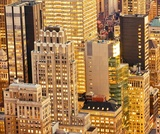Rating
0.0 stars

Surface Area and Volume

Type of Unit: Conceptual

Prior Knowledge

Students should be able to:

Identify rectangles, parallelograms, trapezoids, and triangles and their bases and heights.
Identify cubes, rectangular prisms, and pyramids and their faces, edges, and vertices.
Understand that area of a 2-D figure is a measure of the figure's surface and that it is measured in square units.
Understand volume of a 3-D figure is a measure of the space the figure occupies and is measured in cubic units.

Lesson Flow

The unit begins with an exploratory lesson about the volumes of containers. Then in Lessons 2–5, students investigate areas of 2-D figures. To find the area of a parallelogram, students consider how it can be rearranged to form a rectangle. To find the area of a trapezoid, students think about how two copies of the trapezoid can be put together to form a parallelogram. To find the area of a triangle, students consider how two copies of the triangle can be put together to form a parallelogram. By sketching and analyzing several parallelograms, trapezoids, and triangles, students develop area formulas for these figures. Students then find areas of composite figures by decomposing them into familiar figures. In the last lesson on area, students estimate the area of an irregular figure by overlaying it with a grid. In Lesson 6, the focus shifts to 3-D figures. Students build rectangular prisms from unit cubes and develop a formula for finding the volume of any rectangular prism. In Lesson 7, students analyze and create nets for prisms. In Lesson 8, students compare a cube to a square pyramid with the same base and height as the cube. They consider the number of faces, edges, and vertices, as well as the surface area and volume. In Lesson 9, students use their knowledge of volume, area, and linear measurements to solve a packing problem.

Subject:
Mathematics
Geometry
Material Type:
Unit of Study
Provider:
Pearson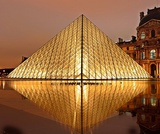Conditional Remix & Share Permitted
CC BY-NC
Rating
0.0 stars

Lesson OverviewStudents use scissors to transform a net for a unit cube into a net for a square pyramid. They then investigate how changing a figure from a cube to a square pyramid affects the number of faces, edges, and vertices and how it changes the surface area and volume.Key ConceptsA square pyramid is a 3-D figure with a square base and four triangular faces.In this lesson, the net for a cube is transformed into a net for a square pyramid. This requires cutting off one square completely and changing four others into isosceles triangles.It is easy to see that the surface area of the pyramid is less than the surface area of the cube, because part of the cube's surface is cut off to create the pyramid. Specifically, the surface area of the pyramid is 3 square units, and the surface area of the cube is 6 square units. Students will be able to see visually that the volume of the pyramid is less than that of the cube.Students consider the number of faces, vertices, and edges of the two figures. A face is a flat side of a figure. An edge is a segment where 2 faces meet. A vertex is the point where three or more faces meet. A cube has 6 faces, 8 vertices, and 12 edges. A square pyramid has 5 faces, 5 vertices, and 8 edges.Goals and Learning ObjectivesChange the net of a cube into the net of a pyramid.Find the surface area of the pyramid.

Subject:
Geometry
Material Type:
Lesson Plan
Provider:
Pearson
09/21/2015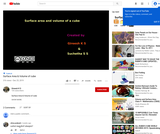Conditional Remix & Share Permitted
CC BY-NC-SA
Rating
5.0 stars

Here they learn Total Surface area, Lateral SUrface ARea and volume of Cube

Subject:
Mathematics
Material Type:
Lesson
Author:
K S Gireesh
Suchetha S S
01/15/2020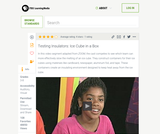Educational Use
Rating
0.0 stars

Watch the ZOOM cast discover which insulator -- newspaper or aluminum foil -- is better at keeping an ice cube frozen longer.

Subject:
Engineering
Education
Chemistry
Physics
Material Type:
Activity/Lab
Provider:
PBS LearningMedia
Provider Set:
PBS Learning Media: Multimedia Resources for the Classroom and Professional Development
Author:
National Science Foundation
WGBH Educational Foundation